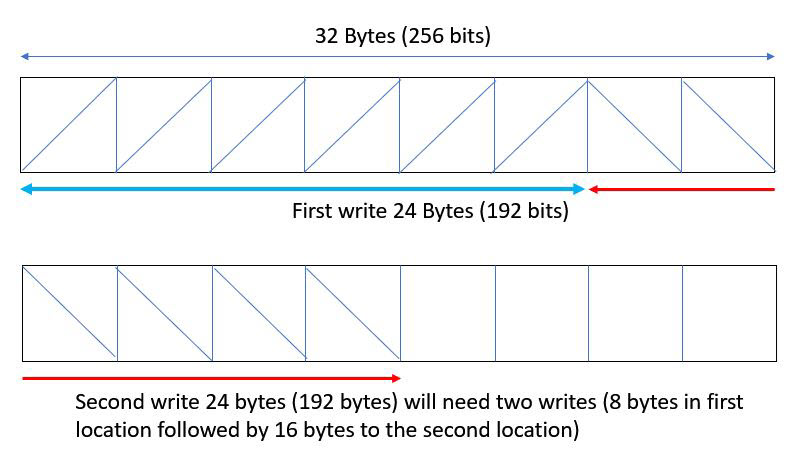# Impact of Struct Size on Pipelining - 2022.1 English

## Vitis High-Level Synthesis User Guide (UG1399)

Document ID
UG1399
Release Date
2022-06-07
Version
2022.1 English

The size of a struct used in a function interface can adversely impact pipelining of loops in that function that have access to the interface in the loop body. Consider the following code example which has two M_AXI interfaces:

``````struct A { /* Total size = 192 bits (32 x 6) or 24 bytes */
int s_1;
int s_2;
int s_3;
int s_4;
int s_5;
int s_6;
};

void read(A *a_in, A buf_out[NUM]) {
for (int i = 0; i < NUM; i++)
{
buf_out[i] = a_in[i];
}
}

void compute(A buf_in[NUM], A buf_out[NUM], int size) {
COMPUTE:
for (int j = 0; j < NUM; j++)
{
buf_out[j].s_1 = buf_in[j].s_1 + size;
buf_out[j].s_2 = buf_in[j].s_2;
buf_out[j].s_3 = buf_in[j].s_3;
buf_out[j].s_4 = buf_in[j].s_4;
buf_out[j].s_5 = buf_in[j].s_5;
buf_out[j].s_6 = buf_in[j].s_6 % 2;
}
}

void write(A buf_in[NUM], A *a_out) {
WRITE:
for (int k = 0; k < NUM; k++)
{
a_out[k] = buf_in[k];
}
}

void dut(A *a_in, A *a_out, int size)
{
#pragma HLS INTERFACE m_axi port=a_in bundle=gmem0
#pragma HLS INTERFACE m_axi port=a_out bundle=gmem1
A buffer_in[NUM];
A buffer_out[NUM];

#pragma HLS dataflow
compute(buffer_in, buffer_out, size);
write(buffer_out, a_out);
}``````

In the above example, the size of struct `A` is 192 bits, which is not a power of 2. As stated earlier in the document, all AXI4 interfaces are by default sized to a power of 2. Vitis HLS will automatically size the two M_AXI interfaces (`a_in` and `a_out`) to be of size 256 - the closest power of 2 to the size of 192 bits (and report in the log file as shown below).

``````INFO: [HLS 214-241] Aggregating maxi variable 'a_out' with compact=none mode in
256-bits (example.cpp:49:0)
INFO: [HLS 214-241] Aggregating maxi variable 'a_in' with compact=none mode in 256-bits
(example.cpp:49:0)``````

This will imply that when writing the struct data out, the first write will write 24 bytes to the first buffer in one cycle but the second write will have to write 8 bytes to the remaining 8 bytes in the first buffer and then write 16 bytes into a second buffer resulting in two writes - as shown in the figure below.

Figure 1. Misaligned Write CyclesThis will cause the II of the WRITE loop in function `write()` to have an II violation since it needs II=2 instead of II=1. Similar behavior will happen when reading and therefore the `read()` function will also have an II violation since it needs II=2. Vitis HLS will issue the following warning for the II violation in function `read()` and `write()`:

``````WARNING: [HLS 200-880] The II Violation in module 'read_r' (loop 'READ'): Unable
to enforce a carried dependence constraint (II = 1, distance = 1, offset = 1) between

WARNING: [HLS 200-880] The II Violation in module 'write_Pipeline_WRITE' (loop 'WRITE'):
Unable to enforce a carried dependence constraint (II = 1, distance = 1, offset = 1)
between bus write operation ('gmem1_addr_write_ln44', example.cpp:44) on port 'gmem1'
(example.cpp:44) and bus write operation ('gmem1_addr_write_ln44', example.cpp:44) on
port 'gmem1' (example.cpp:44).``````

The way to fix such II issues is to pad struct `A` with 8 additional bytes such that you are always writing 256 bits (32 bytes) at a time or by using the other alternatives shown in the table below. This will allow the scheduler to schedule the reads/writes in the READ/WRITE loop with II=1.

Table 1. Struct Alignment
Code Block Description
``````struct A {
int s_1;
int s_2;
int s_3;
int s_4;
int s_5;
int s_6;
};``````
Defines the total size of the struct as 256 bits (32 x 8) or 32 bytes, by adding required padding elements.
``````struct A {
int s_1;
int s_2;
int s_3;
int s_4;
int s_5;
int s_6;
} __attribute__ ((aligned(32)));
``````
Uses the standard `__aligned__` attribute.
``````struct alignas(32) A {
int s_1;
int s_2;
int s_3;
int s_4;
int s_5;
int s_6;
}``````
Uses the C++ standard `alignas` type specifier to specify custom alignment of variables and user defined types.